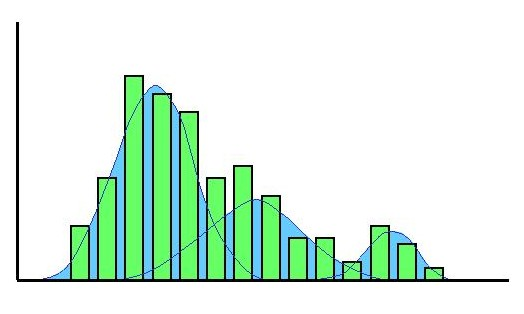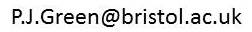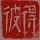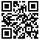Mathematics home Statistics home Postgrad opportunities Statistics clinicPeter Green Research Papers Collaborators Software Books Links Personal Statistical Science Editorial policy Future papers Instructions for authors SuSTaIn ACEMS Past Earth Network Royal Statistical Society International Society for Bayesian Analysis Royal Society

PJG Home / Software### Nmix

-- a program for Bayesian analysis of univariate normal mixtures, implementing the approach of Richardson and Green, Journal of the Royal Statistical Society, B, 59, 731-792 (1997).
If you download the files, please send a message to me at, with your email address and any comments. I can then keep you informed of any updates.

```
INSTALLATION

The package distributed consists of:
main source file        Nmix.f
auxiliary routines in
fortran and C     algama.f gauss4.f pnorm.f rgamma.f sd.c
makefile                Makefile
example data files      enz.dat galx.dat lnacid.dat

It is provided as both a gzipped tarfile and as a zip archive.
In the former, the files all have Unix-style newline characters,
and in the latter Dos-style line endings. The makefiles should be
appropriate for Unix and Dos respectively.

For a free Unix-like shell that runs under Windows, try Cygwin
(www.cygwin.com).

The program has been run successfully:
using f77 and cc under Solaris on Sun workstations
using GNU compilers under Linux on Intel processors
using GNU compilers and Cygwin under Windows on Intel PCs
on Compaq Alpha workstations (with edits: iseed in the main
program has to be declared as integer*8, and the random
number routines are sensitive to rounding error unless
variables are declared as double precision)
on IBM workstations using f77 and cc under AIX 4.3.2 (with edit:
remove the -DSUNF option from cc in the Makefile)

---------------------------------------------

RUNNING

Nmix is a Fortran program for Unix-like systems, implementing
the methodology for univariate normal mixture
analysis described in Richardson and Green
correction in J. R. Statist. Soc. B, 1998, 60, 661).
These notes assume familiarity with this paper.

The program reads a single main data file, and produces
multiple output text files summarising the posterior
distribution of the model, for subsequent analysis
and display (R or Splus are good choices for these
tasks). (Only very limited summary information
is computed by this program itself.)

The run of the program is controlled by options, switches
and parameter settings that have sensible default values,
and can be set by command line arguments in Unix shell
style. For example, the runs on the Enzyme dataset reported
in Section 4.1 of the paper, with run-length of 100000,
burn-in of 100000, and minimal output options could be
replicated by:

Nmix -n100000 -nb100000 enz

The runs described in Section 5.1.1 leading to Fig. 6(a)
would use the -b option, -b0.08 -b0.02 and -b0.005
respectively (given that range-based hyperparameters
are in use (see Section 2.4 of paper), by default).

The principal options are as follows, listed in
several main groups. In this list, N denotes
a numerical value passed in as a parameter of the model
or sampler, other options are logical settings
that are by default off, turned on by specifying the
option.

1. Model options

-prior      disregard the likelihood and the data (except possibly
in setting prior settings, etc.), thus computing the
-fix        suppress dimension-changing moves, thus fixing the
number of mixture components (to the value set by the
-ki option below).

2. Parameter settings

defaults, including use of range-based hyperparameters,
are as used in Section 4.1.

-norange    do NOT use range-based hyperparameters
(default: use them)
-lN         set lambda (hyperparameter for k) (default -1)
lambda=-1: Uniform
lambda=0: p(k)=1/k
lambda>0: Poisson(lambda)
-dN         set delta (hyperparameter for weights) (default 1)
-xN         set xi (hyperparameter for means) (default 0)
-kN         set kappa (hyperparameter for means) (default 1)
negative value signifies Gamma(e,f)
-spN        set s (spacing parameter in prior for means)
(default 1)
(see rejoinder to discussion of paper, page 786)
-aN         set alpha (hyperparameter for variances) (default 2)
-bN         set beta (hyperparameter for variances) (default
Gamma(g,h))
-gN         set g (hyperparameter for beta) (default 0.2)
-hN         set h (hyperparameter for beta) (default 10)
-eN         set e (hyperparameter for kappa) (default 0)
-fN         set f (hyperparameter for kappa) (default 0)

The upper limit kmax of the number of components
is hard-wired equal to 30 in the program - it can
be changed by editing line 1 of Nmix.f (where
it is termed ncmax)

3. Monte Carlo sampler options

-nN         number of sweeps (default 10000)
-nbN        length of burn-in (default 0)
-kiN        initial number of components (default 1)
-noempty    do NOT use empty-component birth-death moves
-seedN      random number seed (default 0, meaning use clock
time to initialise). In the log file for the run,
the seed actually used is printed, and the run
can be repeated with the same random numbers by
specifying that value in this option on the
subsequent run.

4. Output options

-pLETTERS   additional output files, if LETTERS includes
d:    .avn .den .dev
c:    .pcl .scl
w:    .wms.*
a:    .z.*
(default: none of these)
a, w, and c each force d as well
See below for contents of these files.
-full       same as -pd
-nsN        sets nsamp: number of (equi-spaced) Monte Carlo
samples dumped in .out file (default 100)
-nspN       sets nspace: spacing in sweeps between successive
records in trace files .ent, .bk, .dev, .wms*,
.z* (default 20)
-debug      turn on verbose output

Input and output file names

The input data should be in a file with extension .dat,
with the sample size n on the first line, and the data
values y_1, y_2, ..., y_n following in free format, with arbitrary
spaces and newlines.

If the input file is NAME.dat, then all output files
are in a subdirectory NAME (which must be created before
running Nmix). All these output files are given names of the
form N.ext, where N is a unique integer assigned by the program
to label successive runs (it chooses the smallest positive integer
such that N.log does not already exist), and .ext signifies the
type of output the file contains.

The extensions and their meanings are:

(a) normal output:

.log        logs information about run, parameter settings,
acceptance rates, etc.
.k          posterior for k, Bayes factors, prior on k,
posterior for kpos, empty-component statistics
and, optionally, average deviances, etc.
.pe         parameter estimates: posterior means for weights,
means and standard deviations for each component,
conditional on each value of k
.out   [*]  sample of output states: k, kpos,
weight, mean, standard deviation and number of
observations assigned, for each component
.bdlog [*]  changes to k or kpos: number of sweep (file not
produced when -fix option set)
.ent [nsp]  k, kpos, entropy

(b) additionally, if beta or kappa are random:

.bk  [nsp]  beta, kappa, k, kpos

(c) optionally, if -debug specified

.db         verbose records of all moves of the sampler

(d) optionally, as determined by -p... options (see above)

.avn        for each k, number of visits to k, average numbers
of observations assigned to each component
.den        posterior expected density, on a grid of 200
equi-spaced
y values (spanning the range of the data, expanded by
5% in each direction): output is grid, density,
conditional
on k=1,2,...,6, and unconditionally
.dev  [nsp] k, kpos, deviance, modified deviance
.pcl        predictive classification: for each k, for each
j=1,2,..,k-1, for each y* on grid (see .den above),
posterior probability that z*=j
.scl        within-sample classification: for each k, for each
j=1,2,..,k-1, for each i = 1,2,...,n, posterior
probability that z(i)=j
.wms.K [nsp]in a separate file for each k, current values for
weights, means and standard deviations of each
component j=1,2,...,k
(thus each sample record is of k consecutive lines)
.z.K  [nsp] in a separate file for each k, current values for
allocations z(i),i=1,...,n

key:  kpos = number of non-empty components
[nsp] - trace files with output dumped every nspace sweeps (see
-nsp option above)
[*]   - other trace files
other output files contain information aggregated over the run.

```

Nmix is free of charge for educational and non-commercial research purposes.

Please report any problems with the code to the author, Peter Green, via Email link

 Professor Peter Green, School of Mathematics, University of Bristol, Bristol, BS8 1TW, UK. Email link Telephone: +44 (0)117 928 7967; Fax: +44 (0)117 928 7999